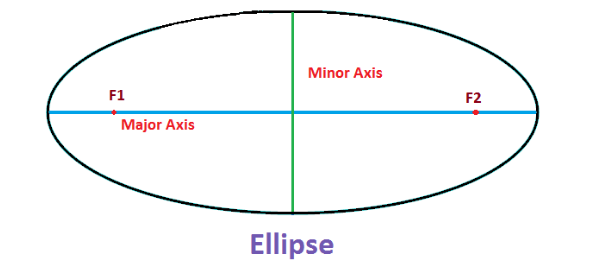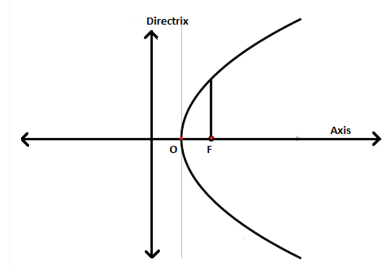# Locus

A locus is a set of points, in geometry, which satisfies a given condition or situation for a shape or a figure. The plural of the locus is loci. The area of the loci is called the region. The word locus is derived from the word location. Before the 20th century, geometric shapes were considered as an entity or place where points can be located or can be moved. But in modern Maths, the entities are considered as the set of points that satisfy the given condition. In this article, let us learn the definition of locus, different locus theorems, and examples with a complete explanation.

## Locus Meaning

In Mathematics, a locus is a curve or other shape made by all the points satisfying a particular equation of the relation between the coordinates, or by a point, line, or moving surface. All the shapes such as circle, ellipse, parabola, hyperbola, etc. are defined by the locus as a set of points.

In real-life you must have heard about the word ‘location’. The word location is derived from the word locus itself. Locus defines the position of something. When an object is situated somewhere, or something happened at a place, is described by locus. For example, the area has become a locus of opposition to the government.

### What is Locus?

A locus is a set of all the points whose position is defined by certain conditions. For example, a range of the Southwest that has been the locus of a number of Independence movements. Here, the locus is defining as the centre of any location.

In Maths, a locus is the set of points represented by a particular rule or law, or equation.

### Locus of Points

The locus of points defines a shape in geometry. Suppose, a circle is the locus of all the points which are equidistant from the centre. Similarly, the other shapes such as an ellipse, parabola, hyperbola, etc. are defined by the locus of the points.

The locus is defined only for curved shapes. These shapes can be regular or irregular.

Locus is not described for the shapes having vertex or angles inside them.

### Locus of a Circle

The set of all points which form geometrical shapes such as a line, a line segment, circle, a curve, etc., and whose location satisfies the conditions is the locus. So, we can say, instead of seeing them as a set of points, they can be seen as places where the point can be located or move.

With respect to the locus of the points or loci, the circle is defined as the set of all points equidistant from a fixed point, where the fixed point is the centre of the circle and the distance of the sets of points from the centre is the radius of the circle. Let us say, P is the centre of the circle and r is the radius of the circle, i.e. the distance from point P to the set of all points or the locus of the points.

## Six Important Locus Theorems

There are six important locus theorems which are popular in geometry. These theorems may be confusing at first reading, but their concepts are actually easy to understand. Let us discuss the six important theorems in detail.

Locus Theorem 1:

The locus at the fixed distance “d” from the point “p” is considered to be a circle with “p” as its center and “d” as its diameter.

This theorem helps to determine the region formed by all the points which are located at the same distance from a single point

Locus Theorem 2:

The locus at a fixed distance “d” from the line “m” is considered as a pair of parallel lines that are located on either side of “m” at a distance “d” from the line  “m”.

This theorem helps to find the region formed by all the points which are located at the same distance from a single line.

Locus Theorem 3:

The locus which is equidistant from the two given points say A and B, are considered as perpendicular bisectors of the line segment that joins the two points.

This theorem helps to determine the region formed by all the points which are located at the same distance from point A and as from point B. The region formed should be the perpendicular bisector of the line segment AB.

Locus Theorem 4:

The locus which is equidistant from the two parallel lines say m1 and m2, is considered to be a line parallel to both the lines m1 and m2 and it should be halfway between them.

This theorem helps to find the region formed by all the points which are at the same distance from the two parallel lines.

Locus Theorem 5:

The locus which is present on the interior of an angle equidistant from the sides of an angle is considered to be the bisector of the angle.

This theorem helps to determine the region formed by all the points which are at the same distance from both sides of an angle. The region should be the angle bisector.

Locus Theorem 6:

The locus which is equidistant from the two intersecting lines say m1 and m2, is considered to be a pair of lines that bisects the angle produced by the two lines m1 and m2.

This theorem helps to find the region formed by all the points which are located at the same distance from the two intersecting lines. The region formed should be a pair of lines that bisect the angle formed.

### Locus Examples in Two Dimensional Geometry

We have already discussed the locus of the points which defines the path for a shape (as explained by a circle). Now let us see some more examples in 2-D geometry or plane geometry.

Perpendicular Bisector:

The set of points that bisects the line, formed by joining two points and are equidistant from two points, is called a perpendicular bisector.

Angle Bisector:

A locus or set of points that bisects an angle and are equidistant from two intersecting lines, which forms an angle, is called an angle bisector.

Ellipse:

Ellipse is defined as the set of points that satisfies the condition where the sum of the distances of two foci points is constant.Parabola:

The set of points or loci, which are equidistant from a fixed point and a line, is called a parabola. The fixed point is the focus and the line is the directrix of the parabola.Hyperbola:

A hyperbola has two focus points, which are equidistant from the centre of the semi-major axis. Hyperbola is defined as the set of points, which satisfies the condition where the absolute value of the difference between the distances to two given foci is a constant.

### Solved Example on Locus

Example:

Find the equation of a point’s locus so that the sum of its distances from (0, -1) and (0,1) is 3. What exactly does the equation mean?

Solution

Suppose P(x, y) be a point on the given locus.

It is given that

A = (0, −1)

B = (0, 1)

From the conditions given,

Distance from Point P to A + Distance from Point P to B = 3

Hence, we can write it as

PA + PB = 3

By rearranging the above equation,

PA = 3 − PB

Now, Squaring both sides of the equation,

PA2=(3−PB)2

PA= 9 + PB− 6PB

x2+(y+1)= 9+x2+(y−1)2−6PB

(y+1)2−(y−1)2−9=−6PB

4y−9 = −6PB

Again, squaring both sides,

16y2−72y+81 = 36PB2

16y2−72y+81 = 36(x2+(y−1)2)

16y2−72y+81 = 36x2+36y2−72y+36

36x2+20y= 45

The above equation is written as follows

(x2/a2)+(y2/b2)=1

Hence, the above equation defines an ellipse.

Therefore, the equation of locus is,

36x2+20y= 45, which is an ellipse.

## Frequently Asked Questions on Locus

Q1

### What is meant by the locus?

In geometry, a locus defines the set of all points whose location is determined by one or more specified constraints.

Q2

### What is meant by the locus of points?

The locus of points is defined as the set of points that satisfy certain properties. For example, a circle is a locus of points.

Q3

### Define the locus of a circle?

The locus of a circle is defined as a set of points on a plane at the same distance from the center point.

Q4

### What is the locus of a straight line?

If the locus is a straight line, then the gradient between any two points on the locus should be equal.

Q5

### What is the equation of locus?

The equation of a locus is defined as a curve that contains the points, whose coordinates satisfy the equation.

Download BYJU’S-The Learning App and get personalized video content explaining the concepts of geometry.